SPSS TUTORIALS BASICS ANOVA REGRESSION FACTOR CORRELATION

# SPSS Median Test for 2 Independent Medians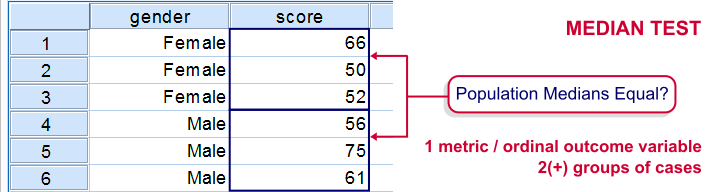The median test for independent medians tests if two or more populations have equal medians on some variable. That is, we're comparing 2(+) groups of cases on 1 variable at a time.

## Rating Car Commercials

We'll demonstrate the median test on adratings.sav. This file holds data on 18 respondents who rated 3 different car commercials on attractiveness. A percent scale was used running from 0 (extremely unattractive commercial) through 100 (extremely attractive).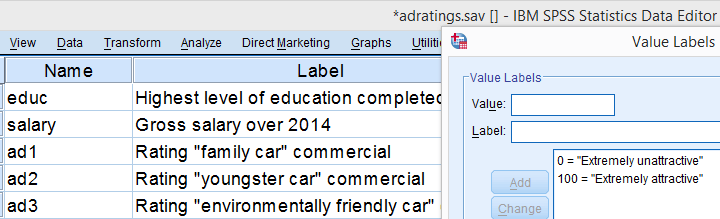## Median Test - Null Hypothesis

A marketeer wants to know if men rate the 3 commercials differently than women. After comparing the mean scores with a Mann-Whitney test, he also wants to know if the median scores are equal. A median test will answer the question by testing the null hypothesis that the population medians for men and women are equal for each rating variable.

## Median Test - Assumptions

The median test makes only two assumptions:

• independent observations (or more precisely, independent and identically distributed variables);
• the test variable is ordinal or metric (that is, not nominal).

## Quick Data Check

The adratings data don't hold any weird values or patterns. If you're analyzing any other data, make sure you always start with a data inspection. At the very least, run some histograms and check for missing values.

## Median Test - Descriptives

Right, we're comparing 2 groups of cases on 3 rating variables. Let's first just take a look at the resulting 6 medians. The fastest way for doing so is running a basic MEANS command.

*Inspect medians for males and females separately.

/cells count mean median.

## Result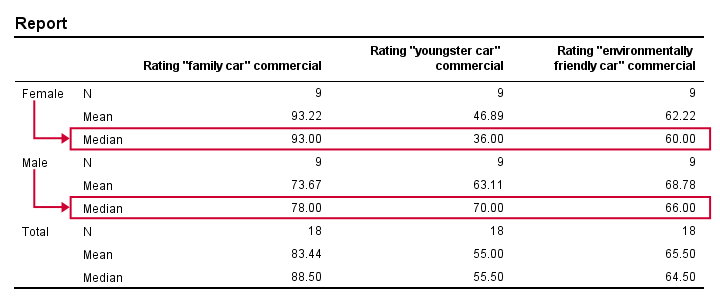Very basically, the “family car” commercial was rated better by female respondents. Males were more attracted to the “youngster car” commercial. The “environmentally friendly car” commercial was rated roughly similarly by both genders -with regard to the medians anyway.
Now, keep in mind that this is just a small sample. If the population medians are exactly equal, then we'll probably find slightly different medians in our sample due to random sampling fluctuations. However, very different sample medians suggest that the population medians weren't equal after all. The median test tells us if equal population medians are credible, given our sample medians.

## Median Test in SPSS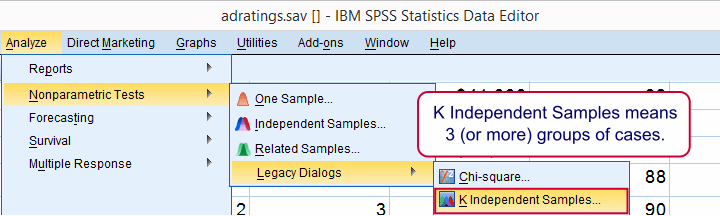Usually, comparing 2 statistics is done with a different test than 3(+) statistics.For example, we use an independent samples t-test for 2 independent means and one-way ANOVA for 3(+) independent means. We use a paired samples t-test for 2 dependent means and repeated measures ANOVA for 3(+) dependent means. We use a McNemar test for 2 dependent proportions and a Cochran Q test for 3(+) dependent proportions. The median test is an exception because it's used for 2(+) independent medians. This is why we select K independent samples instead of 2 independent samples for comparing 2 medians.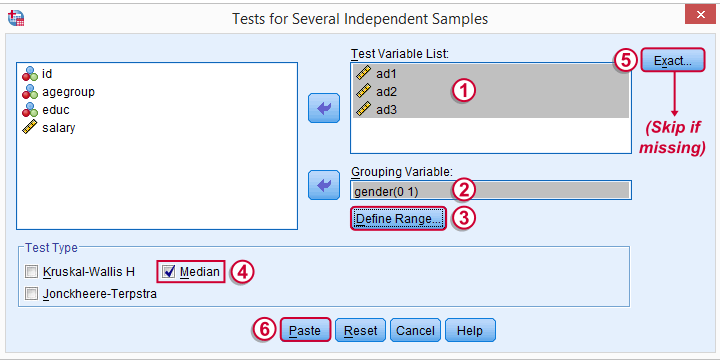The button may be absent, depending on your SPSS license. If it's present, fill it out as below.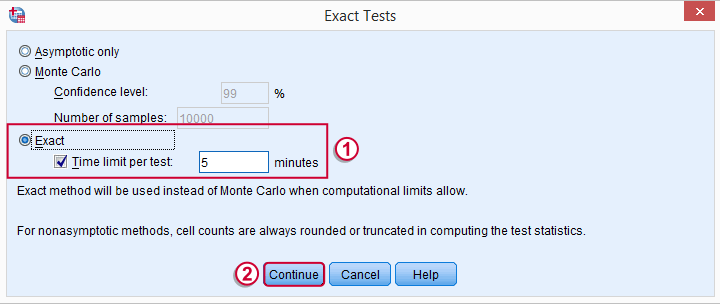## SPSS Median Test Syntax

Completing these steps results in the syntax below (you'll have an extra line if you selected the exact test).

*Basic median test for independent medians.

NPAR TESTS
/MISSING ANALYSIS.

## Median Test - How it Basically Works

Before inspecting our output, let's first take a look at how the test basically works for one variable.2 The median test first computes a variable’s median, regardless of the groups we're comparing. Next, each case (row of data values) is flagged if its score > the pooled median. Finally, we'll see if scoring > the median is related to gender with a basic crosstab.You can pretty easily run these steps yourself with AGGREGATE, IF and CROSSTABS.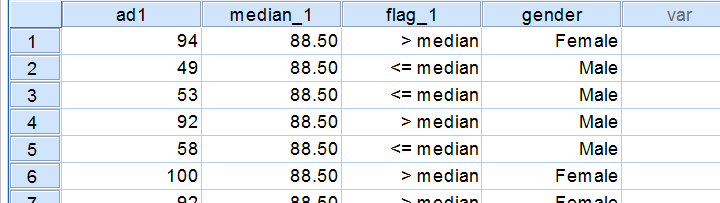## Median Test Output - Crosstabs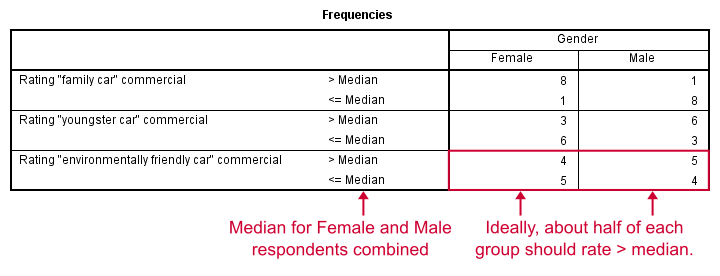Note that these results are in line with the medians we ran earlier. The result for our “family car” commercial is particularly striking: 8 out of 9 respondents who score higher than the (pooled) median are female.

## Median Test Output - Test Statistics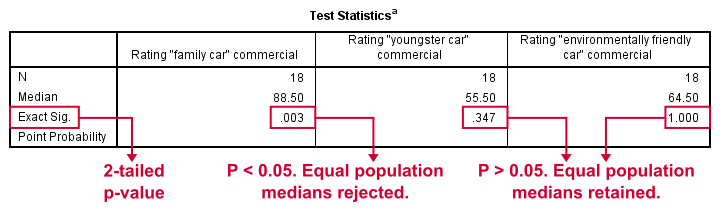So are these normal outcomes if our population medians are equal across gender? For our first commercial, p = 0.003, indicating a chance of 3 in 1,000 of observing this outcome. Since p < 0.05, we conclude that the population medians are not equal for the “family car” commercial.
The other two commercials have p-values > 0.05 so these findings don't refute the null hypothesis of equal population medians.
So that's basically it for now. However, we would like to discuss the p-values into a little more detail for those who are interested.

## Asymp. Sig.

In this example, we got exact p-values. However, when running this test on larger samples you may find “Asymp. Sig.” in your output. This is an approximate p-value based on the chi-square statistic and corresponding df (degrees of freedom). This approximation is sometimes used because the exact p-values are cumbersome to compute, especially for larger sample sizes.

## Exact P-Values

So where do the exact p-values come from? How do they relate to the contingency tables we saw here? Well, the frequencies in the left upper cells follow a hypergeometric distribution.1 Like so, the figure below shows where the second p-value of 0.347 comes from.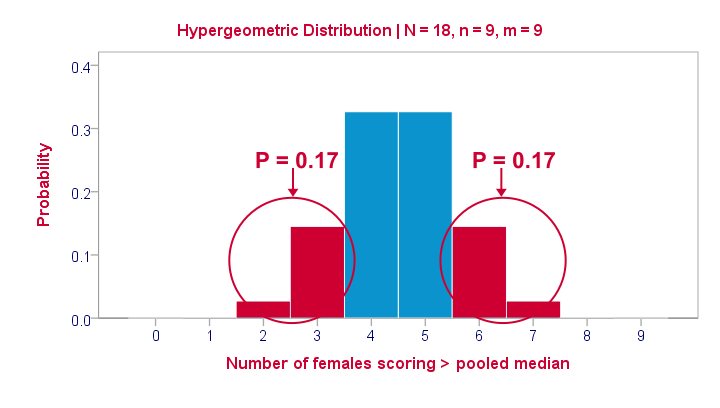Under the null hypothesis -gender and scoring > the median are independent- the most likely outcome is 4 or 5, each with a probability around 0.33. The probability of 3 or fewer is roughly 0.17. This is our one-tailed p-value. Our two-tailed p-value takes into account the probability of 0.17 for finding a value of 6 or more because this would also contradict our null hypothesis.
The graph also illustrates why the two-tailed p-value for our third test is 1.000: the probability of 4 or fewer and 5 or more covers all possible outcomes. Regarding our first test, the probability of 1 or fewer and 8 or more is close to zero (precisely: 0.003).

## Median Test with CROSSTABS

Right, so the previous figure explains how exact p-values are based on the hypergeometric distribution. This procedure is known as Fisher’s exact test and you may have seen it in SPSS CROSSTABS output when running a chi-square independence test. And -indeed- you can obtain the exact p-values for our independent medians test from CROSSTABS too. In fact, you can even compute them as a new variable in your data with compute p2 = 2* cdf.hyper(3,18,9,9).
execute.
which returns 0.347, the p-value for our second commercial.

## References

1. Siegel, S. & Castellan, N.J. (1989). Nonparametric Statistics for the Behavioral Sciences (2nd ed.). Singapore: McGraw-Hill.
2. Van den Brink, W.P. & Koele, P. (2002). Statistiek, deel 3 [Statistics, part 3]. Amsterdam: Boom.

# Tell us what you think!

*Required field. Your comment will show up after approval from a moderator.

# THIS TUTORIAL HAS 2 COMMENTS:

•### By Simon Namnyak on April 27th, 2020

Excellent tutorial. Can Microsoft Excel 365 perform the median test for independent medians tests if two or more populations have equal medians on some variable? That is we're comparing 2(+) groups of cases on 1 variable?

•### By Ruben Geert van den Berg on April 28th, 2020

Hi Simon!

The approximate significance should be very doable as its technically just a chi-square independence test after some data manipulation.

The exact significance would be much harder, though. If you're unwilling to purchase SPSS, try Googling for JASP. This is a free alternative that's just as easy to use. I'm not sure if it includes a (correctly implemented) median test, it may be under ANOVA or "t-test" or something.

Hope that helps!

SPSS tutorials